## Video Lesson

Solve any problem involving stoichiometry using 5 simple steps.

The best way to learn this topic is by working through practice problems. We will look at solving one practice question in this post.

Watch the video for detailed step-by-step explanation of this and another question.

## Question

Calculate the mass of NaCl produced when 2g of sodium reacts with excess chlorine.

## STEP 1 : Write a balanced chemical equation

The first step is to determine the balanced chemical equation of the reaction.
Identify the reactants and products from the question.

From Question 1:
Reactants – sodium and chlorine
Products – sodium chloride

Therefore, the chemical equation would be:

Na (s) + Cl2 (g) → NaCl (s)

Now we need to balance the number of atoms of each element on the left and right sides of the chemical equations.

Left:
Na – 1
Cl – 2

Right:
Na – 1
Cl – 1

To balance the number of chlorine atoms, we need to add “2” in front of NaCl.

Na (s) + Cl2 (g) → 2NaCl (s)

Now we have:

Left:
Na – 1
Cl – 2

Right:
Na – 2
Cl – 2

To balance the number of sodium atoms, we need to add “2” in front of Na.

2Na (s) + Cl2 (g) → 2NaCl (s)

Now we have:

Left:
Na – 2
Cl – 2

Right:
Na – 2
Cl – 2

Now the chemical equation is finally balanced. We have the same number of sodium and chlorine atoms on both sides of the chemical equation.

## Question

Calculate the mass of NaCl produced when 2g of sodium reacts with excess chlorine.

## STEP 2 :  Find mole ratio of species involved

2Na (s) + Cl2 (g) → 2NaCl (s)

Now that we have a balanced chemical equation, we can analyse it quantitatively.

• The number in front of each species is known as the coefficient of the species.
• These coefficients tell us the ratio of moles of the species involved in the chemical reaction.
• From the equation we know that:

2 mol of sodium reacts with 1 mol of chlorine to produce 2 mol of sodium chloride

When deciding which ratio of moles to find, we need to carefully examine the question and look for two things:

• Quantity of species to be found (mass of NaCl)
• Quantity of species given (2g of sodium)

Now we know that we need to find the ratio of moles of sodium chloride, NaCl to sodium, Na.

From the quantitative analysis we did earlier, we know that:

moles of NaCl : moles of Na

= 2 : 2

Both “2“s are the coefficients of each species in the balanced chemical equation.

2Na (s) + Cl2 (g) → 2NaCl (s)

We can simplify this by dividing both numbers by 2 to get:

moles of NaCl : moles of Na

= 2 : 2

= 1 : 1

## Question

Calculate the mass of NaCl produced when 2g of sodium reacts with excess chlorine.

## STEP 3 : Calculate actual number of moles of species with given quantity

• The question tells us that 2g of sodium has reacted.
• This is the given quantity (mass of sodium).
• We can use this quantity to find the number of moles of sodium reacted.

There are four equations for number of moles which must be committed to memory: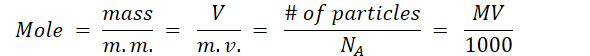m. m. = molar mass
m. v. = molar volume
# of particles = number of particles
NA = Avogardro’s constant = 6.02 x 1023
M = molarity
V = volume

Since we are given the mass of sodium, we would choose the mole formula that includes mass in it: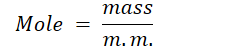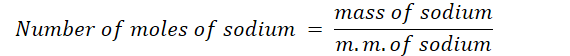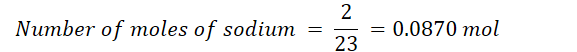## Question

Calculate the mass of NaCl produced when 2g of sodium reacts with excess chlorine.

## STEP 4 : Calculate actual number of moles of species with unknown quantity.

Now that we have the actual number of moles of sodium, we can use the mole ratio we determined in STEP 2 to calculate the actual number of moles of sodium chloride formed:

moles of NaCl : moles of Na
= 1 : 1
= x : 0.0870

Using the fraction form we have: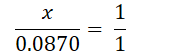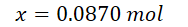## Question

Calculate the mass of NaCl produced when 2g of sodium reacts with excess chlorine.

## STEP 5 : Calculate unknown quantity

From STEP 4, we have the number of moles of sodium chloride formed.
Since we need to find the mass of sodium chloride formed, we should choose the formula that relates mass to number of moles: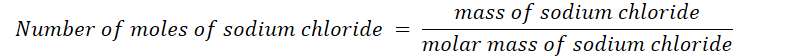The molar mass of sodium chloride has the same value as its relative formula mass.
This can be found by adding the relative atomic mass of all the ions in the formula unit NaCl: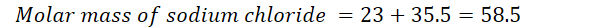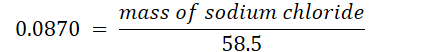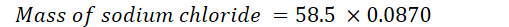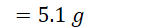Using these 5 steps and the four mole formulas, you can find any quantity (mass/volume/number of particles/molarity) of a species as long as the quantity of another species in the chemical equation is given.

## Practice Questions

1. Calculate the volume of oxygen gas needed at RTP to react with 4g of lithium to form lithium oxide.
Ans: 3.43 dm3
2. Calculate the number of atoms of magnesium that will produce 3.5g of zinc when magnesium reacts with zinc chloride to form zinc and magnesium chloride.
Ans: 3.24 x 1022 atoms
3. Calculate the volume of carbon dioxide produced at RTP when 8.4g of carbon is reacted with excess iron(III) oxide to form iron and carbon dioxide.
Ans: 16.8
dm3

60108280139

Scroll to Top